# Selina Solutions Concise Mathematics Class 6 Chapter 26: Triangles Exercise 26(A)

Selina Solutions Concise Mathematics Class 6 Chapter 26 Triangles Exercise 26(A) consists of problems on determining the angle of the given set of triangles. A triangle is defined as a closed figure which has three sides and vertices. The main objective of preparing exercise wise solutions is to develop a strong foundation of fundamental concepts discussed under this exercise. These concepts are vital as it will be continued in higher class as well. In order to obtain a clear conceptual knowledge, students can access Selina Solutions Concise Mathematics Class 6 Chapter 26 Triangles Exercise 26(A) PDF, from the links which are provided here

## Selina Solutions Concise Mathematics Class 6 Chapter 26: Triangles Exercise 26(A) Download PDF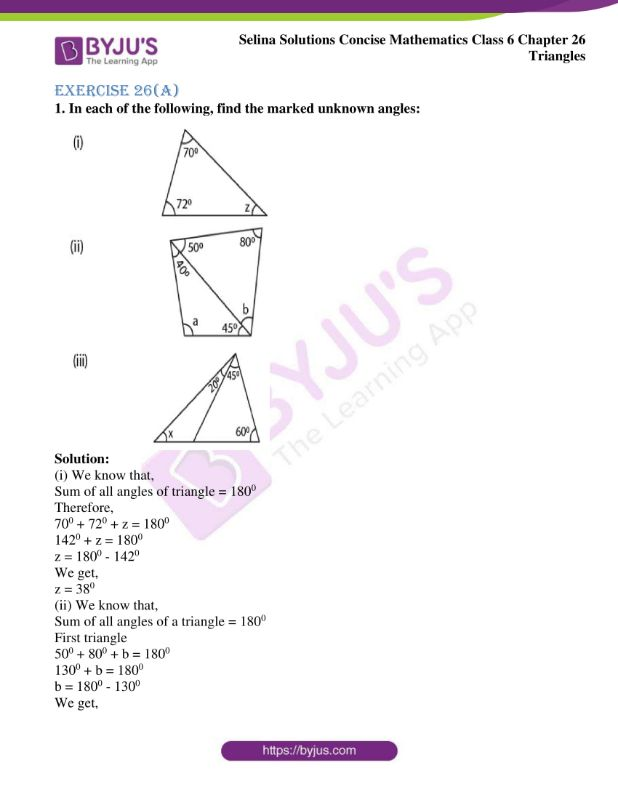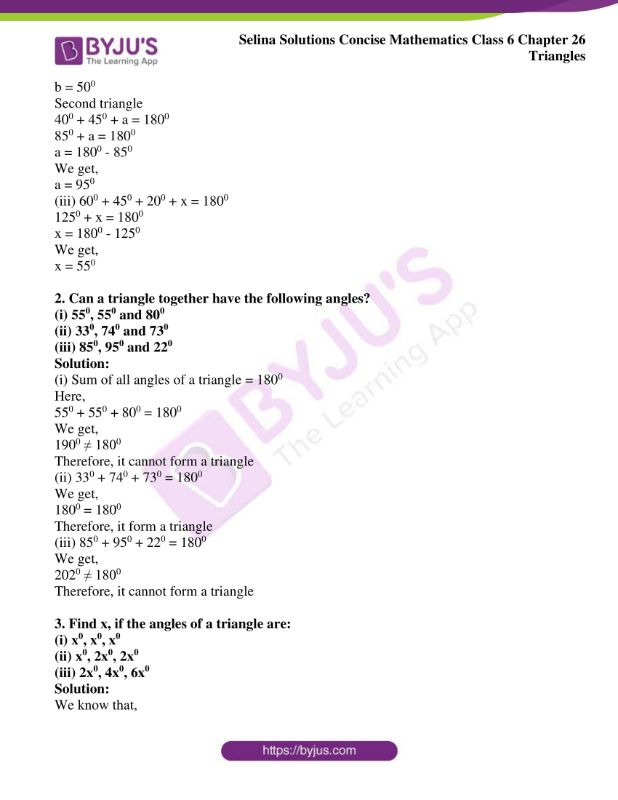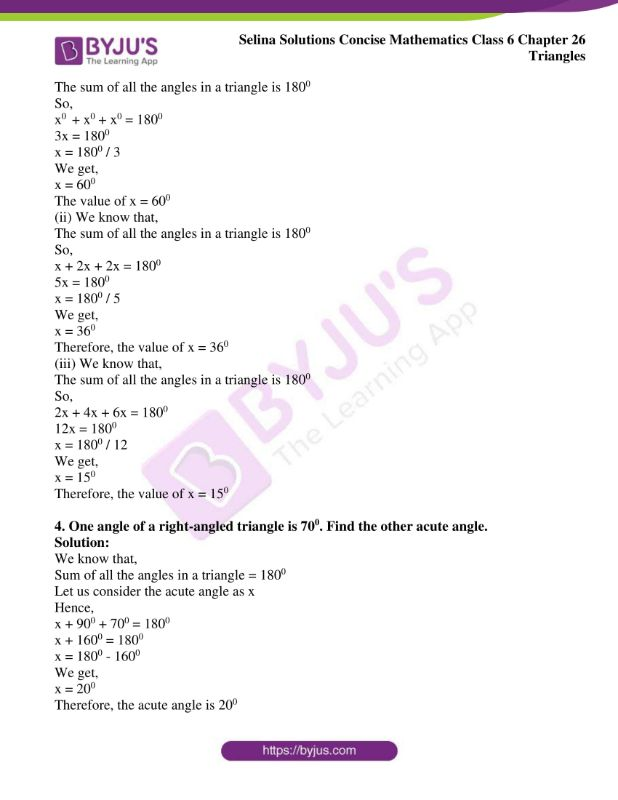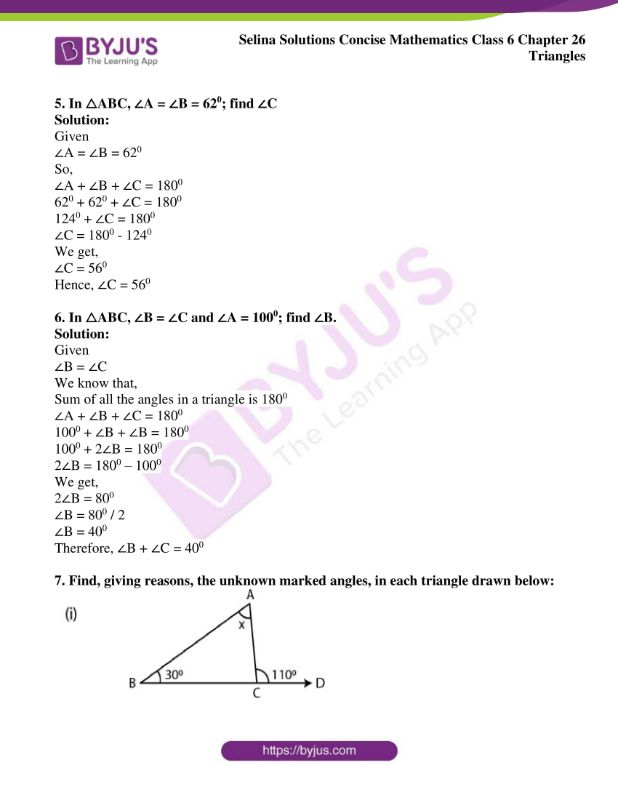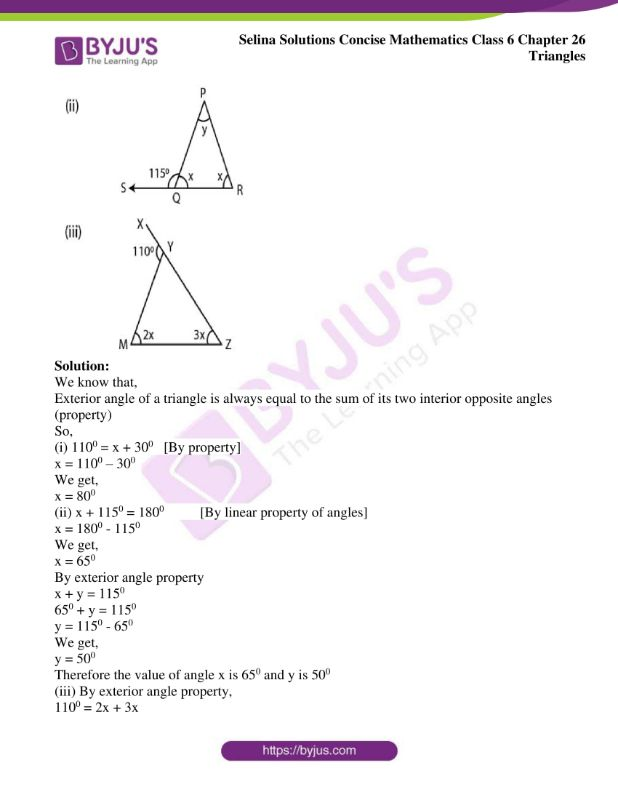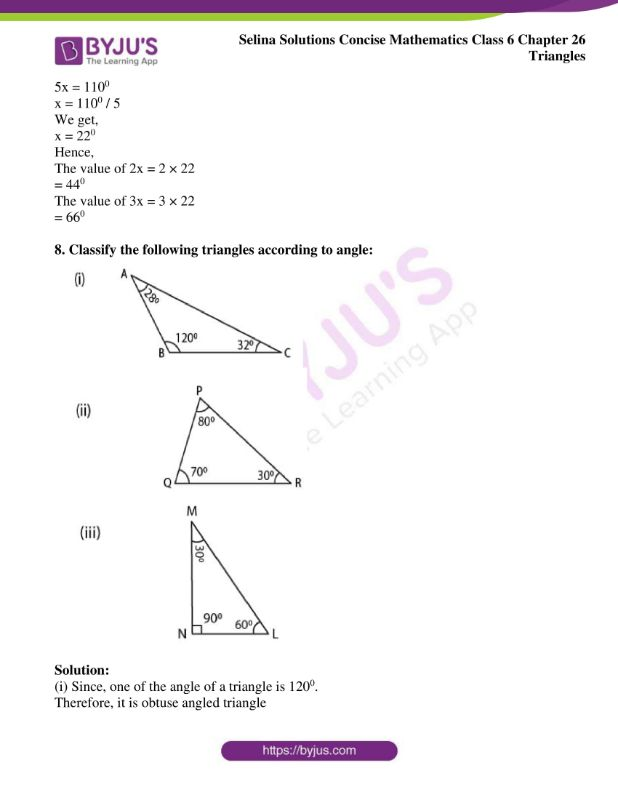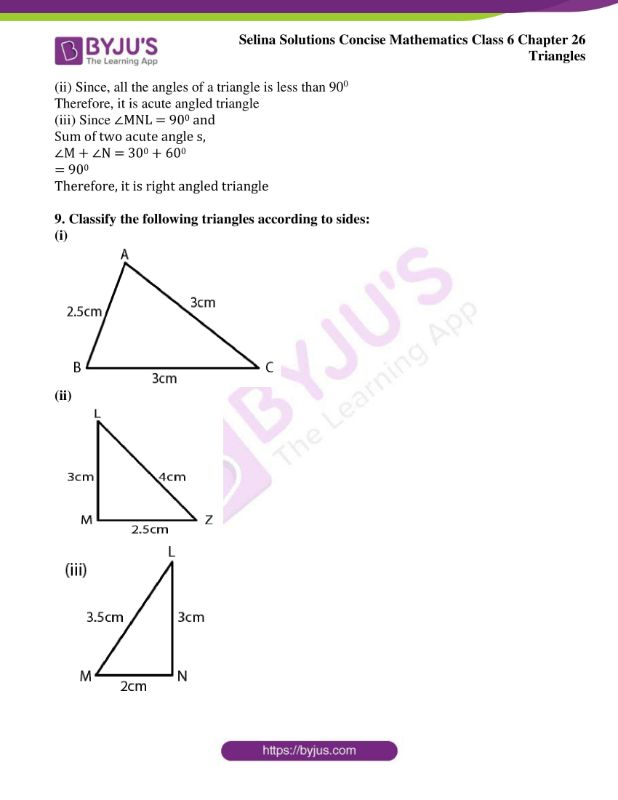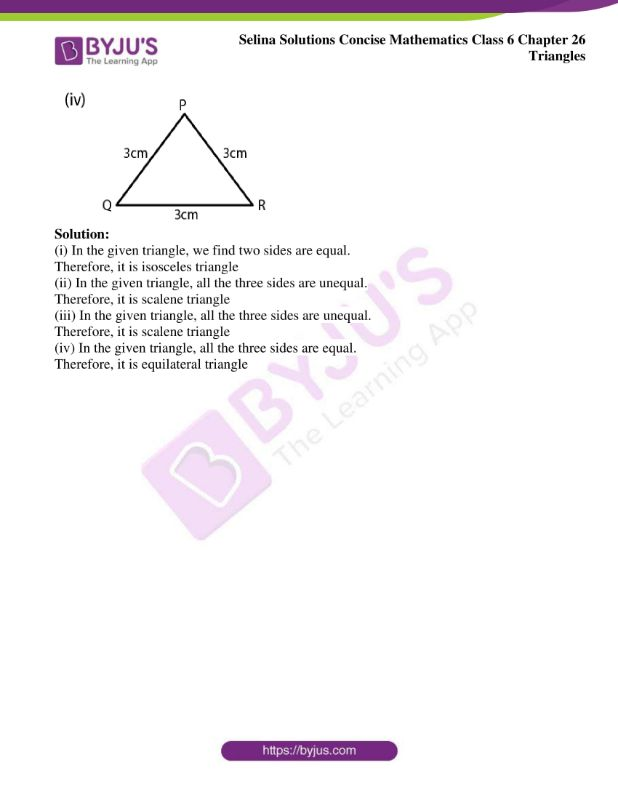### Access Selina Solutions Concise Mathematics Class 6 Chapter 26: Triangles Exercise 26(A)

Exercise 26(A)

1. In each of the following, find the marked unknown angles: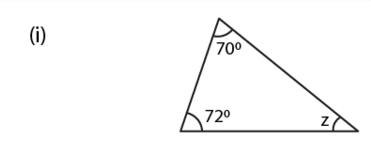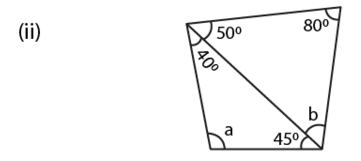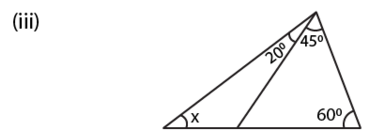Solution:

(i) We know that,

Sum of all angles of triangle = 1800

Therefore,

700 + 720 + z = 1800

1420 + z = 1800

z = 1800 – 1420

We get,

z = 380

(ii) We know that,

Sum of all angles of a triangle = 1800

First triangle

500 + 800 + b = 1800

1300 + b = 1800

b = 1800 – 1300

We get,

b = 500

Second triangle

400 + 450 + a = 1800

850 + a = 1800

a = 1800 – 850

We get,

a = 950

(iii) 600 + 450 + 200 + x = 1800

1250 + x = 1800

x = 1800 – 1250

We get,

x = 550

2. Can a triangle together have the following angles?

(i) 550, 550 and 800

(ii) 330, 740 and 730

(iii) 850, 950 and 220

Solution:

(i) Sum of all angles of a triangle = 1800

Here,

550 + 550 + 800 = 1800

We get,

1900 ≠ 1800

Therefore, it cannot form a triangle

(ii) 330 + 740 + 730 = 1800

We get,

1800 = 1800

Therefore, it form a triangle

(iii) 850 + 950 + 220 = 1800

We get,

2020 ≠ 1800

Therefore, it cannot form a triangle

3. Find x, if the angles of a triangle are:

(i) x0, x0, x0

(ii) x0, 2x0, 2x0

(iii) 2x0, 4x0, 6x0

Solution:

We know that,

The sum of all the angles in a triangle is 1800

So,

x0 + x0 + x0 = 1800

3x = 1800

x = 1800 / 3

We get,

x = 600

The value of x = 600

(ii) We know that,

The sum of all the angles in a triangle is 1800

So,

x + 2x + 2x = 1800

5x = 1800

x = 1800 / 5

We get,

x = 360

Therefore, the value of x = 360

(iii) We know that,

The sum of all the angles in a triangle is 1800

So,

2x + 4x + 6x = 1800

12x = 1800

x = 1800 / 12

We get,

x = 150

Therefore, the value of x = 150

4. One angle of a right-angled triangle is 700. Find the other acute angle.

Solution:

We know that,

Sum of all the angles in a triangle = 1800

Let us consider the acute angle as x

Hence,

x + 900 + 700 = 1800

x + 1600 = 1800

x = 1800 – 1600

We get,

x = 200

Therefore, the acute angle is 200

5. In △ABC, ∠A = ∠B = 620; find ∠C

Solution:

Given

∠A = ∠B = 620

So,

∠A + ∠B + ∠C = 1800

620 + 620 + ∠C = 1800

1240 + ∠C = 1800

∠C = 1800 – 1240

We get,

∠C = 560

Hence, ∠C = 560

6. In △ABC, ∠B = ∠C and ∠A = 1000; find ∠B.

Solution:

Given

∠B = ∠C

We know that,

Sum of all the angles in a triangle is 1800

∠A + ∠B + ∠C = 1800

1000 + ∠B + ∠B = 1800

1000 + 2∠B = 1800

2∠B = 1800 – 1000

We get,

2∠B = 800

∠B = 800 / 2

∠B = 400

Therefore, ∠B + ∠C = 400

7. Find, giving reasons, the unknown marked angles, in each triangle drawn below: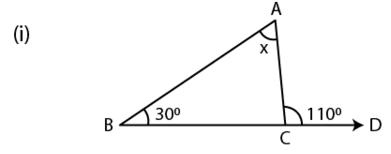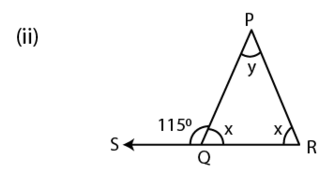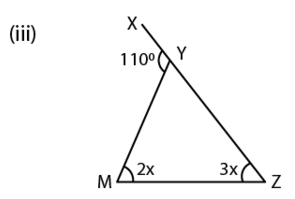Solution:

We know that,

Exterior angle of a triangle is always equal to the sum of its two interior opposite angles (property)

So,

(i) 1100 = x + 300 [By property]

x = 1100 – 300

We get,

x = 800

(ii) x + 1150 = 1800 [By linear property of angles]

x = 1800 – 1150

We get,

x = 650

By exterior angle property

x + y = 1150

650 + y = 1150

y = 1150 – 650

We get,

y = 500

Therefore the value of angle x is 650 and y is 500

(iii) By exterior angle property,

1100 = 2x + 3x

5x = 1100

x = 1100 / 5

We get,

x = 220

Hence,

The value of 2x = 2 × 22

= 440

The value of 3x = 3 × 22

= 660

8. Classify the following triangles according to angle: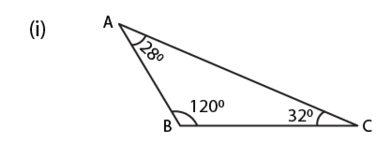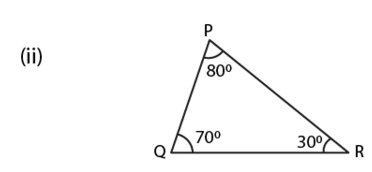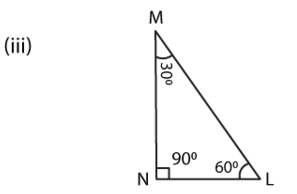Solution:

(i) Since, one of the angle of a triangle is 1200.

Therefore, it is obtuse angled triangle

(ii) Since, all the angles of a triangle is less than 900

Therefore, it is acute angled triangle

(iii) Since ∠MNL = 900 and

Sum of two acute angle s,

∠M + ∠N = 300 + 600

= 900

Therefore, it is right angled triangle

9. Classify the following triangles according to sides:

(i)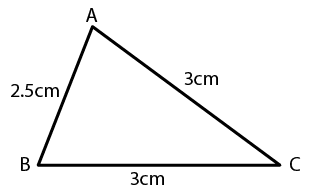(ii)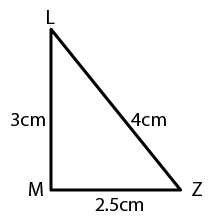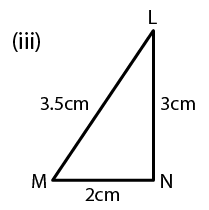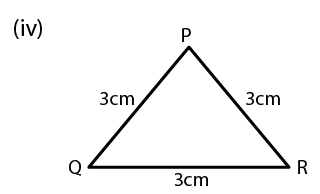Solution:

(i) In the given triangle, we find two sides are equal.

Therefore, it is isosceles triangle

(ii) In the given triangle, all the three sides are unequal.

Therefore, it is scalene triangle

(iii) In the given triangle, all the three sides are unequal.

Therefore, it is scalene triangle

(iv) In the given triangle, all the three sides are equal.

Therefore, it is equilateral triangle